## Quantization for probability measures in the Prokhorov metric

 Title Quantization for probability measures in the Prokhorov metric Publication Type Journal Article Year of Publication 2009 Authors Harald Luschgy, and Siegfried Graf Journal Theory Probab. Appl. Volume 53 Pagination 216-241 Keywords asymptotic quantization error, empirical measures, entropy, Ky Fan metric, multidimensional quantization, optimal quantizers, Prokhorov metric, quantization dimension Abstract For a probability distribution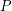on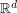and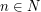consider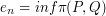, wheredenotes the Prokhorov metric and the infimum is taken over all discrete probabilities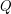with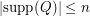. We study solutionsof this minimization problem, stability properties, and consistency of empirical estimators. For some classes of distributions we determine the exact rate of convergence to zero of theth quantization error en as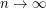.